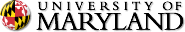An Integral Equation Method for Epitaxial Step-flow Growth Simulations

CSIC Building (#406), Seminar Room 4122.
Directions: home.cscamm.umd.edu/directions

### An Integral Equation Method for Epitaxial Step-flow Growth Simulations

Professor Jingfang Huang

University of North Carolina, Chapel Hill

Abstract:   In this talk, we describe an integral equation approach for simulating diffusion problems with moving interfaces. The solutions are represented as moving layer potentials where the unknowns are only defined on the interfaces. The resulting integro-differential equation (IDE) system is solved using Krylov deferred correction (KDC) techniques developed for general differential algebraic equations (DAEs), and the time dependent potentials are evaluated efficiently using fast convolution algorithms. The numerical solver is applied to the BCF model for the epitaxial step-flow growth of crystals, for which the solutions are calculated accurately instead of using quasi-static approximations. Numerical results in 1+1 dimensions are compared with available results in the literature.UM Home | Directories | Calendar Maintained by CSCAMM Direct questions and comments to webmaster CSCAMM is part of the College of Computer, Mathematical & Natural Sciences (CMNS)